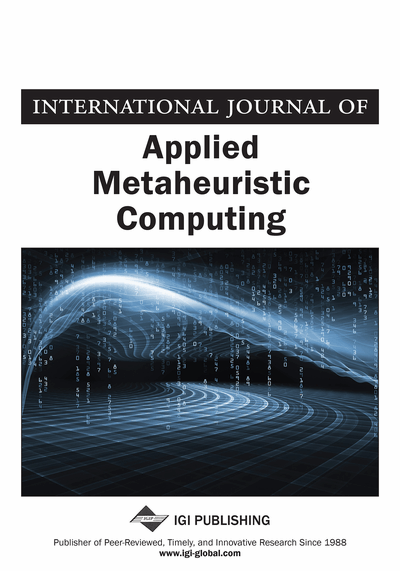# A Hybrid Simulated Annealing and Simplex Method for Fixed-Cost Capacitated Multicommodity Network Design

Masoud Yaghini (Iran University of Science and Technology, Iran), Mohammad Karimi (Iran University of Science and Technology, Iran), Mohadeseh Rahbar (Iran University of Science and Technology, Iran) and Rahim Akhavan (Kermanshah University of Technology, Kermanshah, Iran)
DOI: 10.4018/jamc.2011100102
Available
\$37.50
No Current Special Offers

## Abstract

The fixed-cost Capacitated Multicommodity Network Design (CMND) problem is a well known NP-hard problem. This paper presents a matheuristic algorithm combining Simulated Annealing (SA) metaheuristic and Simplex method for CMND problem. In the proposed algorithm, a binary array is considered as solution representation and the SA algorithm manages open and closed arcs. Several strategies for opening and closing arcs are proposed and evaluated. In this matheuristic approach, for a given design vector, CMND becomes a Capacitated Multicommodity minimum Cost Flow (CMCF) problem. The exact evaluation of the CMCF problem is performed using the Simplex method. The parameter tuning for the proposed algorithm is done by means of design of experiments approach. The performance of the proposed algorithm is evaluated by solving different benchmark instances. The results of the proposed algorithm show that it is able to obtain better solutions in comparison with previous methods in the literature.
Article Preview
Top

## Introduction

The Network Design Problem (NDP) is one of the important problems in combinatorial optimization. The objective of NDP is to find a minimum cost network on the available arcs for each commodity which satisfy flows of commodities. Network design models have numerous applications in various fields such as transportation (Crainic et al., 1989; Magnanti & Wong, 1984) telecommunication (Gendron et al., 1998; Minoux, 1989, 2001) and distribution planning (Jayaraman & Ross, 2002).

The fixed-cost Capacitated Multicommodity Network Design (CMND) problem is one type of the network design models. In this model, multiple commodities such as goods, data, people, etc., must be routed between different points of origin and destination on the limited capacity arcs. In this problem, in addition to a unit cost (variable cost), a fixed cost is usually added due to the opening cost for the first time the arc is used (Ahuja et al., 1993). CMND problem seeks a network with minimum cost which satisfies demands of commodities. This minimum cost is the sum of the fixed and variable costs (Crainic, 2000).

Network design problems can easily be stated but solving them is too difficult (Balakrishnan et al., 1997). There are effective exact solution methods for uncapacitated network design problem. In exact algorithms, finding optimal solution is guaranteed. Benders decomposition and branch-and-bound methods have been the two most effective ones for this type of problem (Magnanti & Wong, 1984). Several surveys on network design models and their exact solution methods can be found in Balakrishnan et al. (1997), Crainic (2000), Frangioni and Gendron (2008), Magnanti and Wong (1984), and Minoux (1989, 2004). In addition, in Minoux (1989), a good survey on models and solution methods for multicommodity capacitated network design problems, especially simplex-based cutting plane and Lagrangian relaxation solution methods has been presented.

Adding capacity to the arcs of network design problem creates more complexity of this problem (Balakrishnan et al., 1997). Furthermore, large-scale network design problems which occur in real world applications are very difficult to solve (Magnanti & Wong, 1984). There are theoretical and empirical evidence that the capacitated network design problems are NP-hard (Balakrishnan et al., 1997; Magnanti & Wong, 1984). That is to say, there is no efficient algorithm which can solve them in polynomial time. As a result, the researchers proposed approximation methods to solve them. However, this type of solution methods cannot guarantee the optimality of the solutions. Metaheuristics that are a kind of approximation methods deal with these problems by introducing systematic rules to escape from local optima (Glover & Kochenberger, 2003).

To deal with CMND problems, several matheuristic algorithms have been proposed in the literature. Matheuristics are heuristic algorithms made by the interoperation of metaheuristics and mathematic programming techniques. This approach has begun to appear regularly in the metaheuristics literature (Blum et al., 2011; Boschetti et al., 2009; Caserta & Voß, 2009).

## Complete Article List

Search this Journal:
Reset
Volume 13: 4 Issues (2022): 2 Released, 2 Forthcoming
Volume 12: 4 Issues (2021)
Volume 11: 4 Issues (2020)
Volume 10: 4 Issues (2019)
Volume 9: 4 Issues (2018)
Volume 8: 4 Issues (2017)
Volume 7: 4 Issues (2016)
Volume 6: 4 Issues (2015)
Volume 5: 4 Issues (2014)
Volume 4: 4 Issues (2013)
Volume 3: 4 Issues (2012)
Volume 2: 4 Issues (2011)
Volume 1: 4 Issues (2010)
View Complete Journal Contents Listing